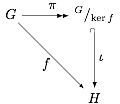# 3rd Grade Math Unit Lesson Ideas: Algebra, Geometry, Measurement, Data & Probability

Page content

## Ugh… Math!

Why teach math? Studying mathematics teaches students necessary life skills; cooking requires knowledge of measurement and fractions, purchasing groceries is difficult without an understanding of money, and being on time is impossible if you cannot tell time. By the end of these math unit lesson plans, third grade students comprehend advanced mathematic concepts.

Here is a guide to planning, writing, and implementing math lessons in the third grade classroom. This outline can be used as-is or customized to suit individual needs and preferences.

Core Components

The core concepts covered in these math activites are algebra, geometry, measurement, data and probability. Some multi-sensory learning experiences are offered via creative lesson plan ideas like baking cookies to develop measuring skills, or doubling recipes to practice with fractions. Other daily lesson plans focus on traditional mathematic concepts.

Algebra Unit

• Whole numbers – rounding, adding, subtracting, estimating, and solving word problems.
• Integers – absolute values, signed integers, multiplying and dividing.
• Fractions – converting, reducing, mixed, and improper fractions.
• Decimals – naming, converting, rounding, mathematical practice.
• Percent – converting percent to decimal, solving word problems.

Geometry Unit

In the geometry weekly unit, the following daily lesson plan ideas are suggested:

• Shape shifting – working with various geometric shapes like polygons, pyramids, and vertices, students create original works of art. Measuring and drawing the shapes onto construction paper is a nice extension to the lesson plan.
• Great Pyramid adventure – working with toothpicks and wood glue, students create a pyramid. Hold a group discussion prior to the hands-on part of the lesson about pyramid structure, construction, and function.
• Boot camp – focus students on the basic elements of geometry like theorems and formulas. Challenge the students to set the formulas to music to make memorization easier. Here is an example; sing to the tune of “Mary had a Little Lamb”: The sum of the three interior angles/of a triangle/ is 180 degrees.
• Match game – Divide the class into two teams. Drill teams on geometry theorems and formulas. The winning team gets a homework pass.
• Wrap-up Day – review the week’s lessons. Assess the depth of learning and reinforce concepts where needed. Use pies to demonstrate the concept of pi.Measurement Unit

Some suggested daily lesson plans for teaching a measurement unit are:

• Got milk? Bring in milk and cookies. Have children practice measuring different amounts of milk or dividing the cookies into fractions, for instance, one-third of a package, or three-fourths. The 3rd graders eat the props at the end of the class.
• Bake cookies – Bake a batch of cookies, and teach measurement skills. Practice the math involved in doubling or tripling a recipe.
• Play I Spy – students spy an object in the classroom, for instance, a picture or book, and use rulers to measure the object in inches and centimeters. Encourage them to record their measurement in a journal, and use the measurement to create a bar graph in the data and probability unit.
• Clocks measure time – work on time telling skills and learning how clocks measure time.
• Wrap-up day – review the math unit lesson plans 3rd grade level for this week. Use oral quizzes to assess comprehension.

3rd Grade Data & Probability Unit

Teaching data collection, graphing, and probability is easier with the use of manipulatives and props like coins and dice. Here are some lesson plans ideas for this unit:

• Bar graphs – using the data gathered during the measurement lesson plan, students create a bar graph.
• Coin toss – using coins, demonstrate and let students do probability experiments.
• Candy graphs – put a predetermined number and colors of candy into a bag. Students graph the results and make probability predictions by pulling candy out of the bag.
• Spinning probabilities – using the spinner from any board game, chart probability and graph results.
• Wrap-up day – review the math unit lesson plans 3rd grade level for data and probability. Use a written quiz to gauge comprehension of subject matter.

These math lesson ideas cover the core mathematical components of algebra, geometry, measurement, data and probability. The third graders understand the concepts and are prepared to advance to more difficult levels of math.

Image Credits: